Updating search results...

# 35 Results

View
Selected filters:
• ratioOnly Sharing Permitted
CC BY-NC-ND
Rating
0.0 stars

BPCC Open Campus - Math 097: Basic Mathematics is a review of basic mathematics skills. Here's what's covered: -fundamental numeral operations of addition, subtraction, multiplication division of whole numbers, fractions, and decimals -ratio and proportion -percent -systems of measurement -an introduction to geometry NOTE: Open Campus courses are non-credit reviews and tutorials and cannot be used to satisfy requirements in any curriculum at BPCC.

Subject:
Education
Mathematics
Material Type:
Assessment
Full Course
Lecture
Lesson
Unit of Study
10/11/2017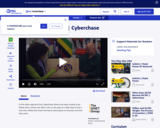Educational Use
Rating
0.0 stars

Bianca visits a bike shop and learns how bicycle gears work in this Cyberchase video segment.

Subject:
Mathematics
Material Type:
Lecture
Provider:
PBS LearningMedia
Provider Set:
PBS Learning Media: Multimedia Resources for the Classroom and Professional Development
Author:
U.S. Department of Education
WNET
09/09/2008Conditional Remix & Share Permitted
CC BY-NC
Rating
0.0 stars

Intro:&nbsp; In this Lesson, students are using a real world example of candy cane sales (presently happening in my school), but could be adopted to be used at other times of the year, with many other specific real world examples that work for your particular situation)

Subject:
Numbers and Operations
Material Type:
Lesson Plan
Author:
Colleen Maguire
12/23/2018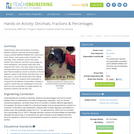Educational Use
Rating
0.0 stars

Students learn about and practice converting between fractions, decimals and percentages. Using a LEGO® MINDSTORMS® NXT robot and a touch sensor, each group inputs a fraction of its choosing. Team members convert this same fraction into a decimal, and then a percentage via hand calculations, and double check their work using the NXT robot. Then they observe the robot moving forward and record that distance. Students learn that the distance moved is a fraction of the full distance, based on the fraction that they input, so if they input ½, the robot moves half of the original distance. From this, students work backwards to compute the full distance. Groups then compete in a game in which they are challenged to move the robot as close as possible to a target distance by inputting a fraction into the NXT bot.

Subject:
Applied Science
Engineering
Mathematics
Technology
Material Type:
Activity/Lab
Provider:
TeachEngineering
Provider Set:
TeachEngineering
Author:
Javed Narain
09/18/2014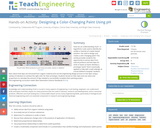Educational Use
Rating
0.0 stars

How can an understanding of pH—a logarithmic scale used to identify the acidity or basicity of a water-based solution—be used to design and create a color-changing paint? This activity provides students the opportunity to extract dyes from natural products and test dyes for acids or bases as teams develop a prototype “paint” that is eventually applied to help with a wall redesign at a local children’s hospital. Students learn about how dyes are extracted from organic material and use the engineering design process to test dyes using a variety of indicators to achieve the right color for their prototype. Students iterate on their dyes and use ratios and proportions to calculate the amount of dye needed to successfully complete their painting project.

Subject:
Applied Science
Chemistry
Engineering
Mathematics
Numbers and Operations
Physical Science
Material Type:
Activity/Lab
Provider:
TeachEngineering
Provider Set:
Activities
Author:
Benjamin McCombs
Carly Monfort
Joseph Duncan
Linda Gillum
Miyong Hughes
01/30/2019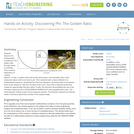Educational Use
Rating
0.0 stars

Students discover the mathematical constant phi, the golden ratio, through hands-on activities. They measure dimensions of "natural objects"—a star, a nautilus shell and human hand bones—and calculate ratios of the measured values, which are close to phi. Then students learn a basic definition of a mathematical sequence, specifically the Fibonacci sequence. By taking ratios of successive terms of the sequence, they find numbers close to phi. They solve a squares puzzle that creates an approximate Fibonacci spiral. Finally, the instructor demonstrates the rule of the Fibonacci sequence via a LEGO® MINDSTORMS® NXT robot equipped with a pen. The robot (already created as part of the companion activity, The Fibonacci Sequence & Robots) draws a Fibonacci spiral that is similar to the nautilus shape.

Subject:
Applied Science
Engineering
Mathematics
Technology
Material Type:
Activity/Lab
Provider:
TeachEngineering
Provider Set:
TeachEngineering
Author:
Nicole Abaid
09/18/2014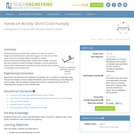Educational Use
Rating
0.0 stars

Student groups are provided with a generic car base on which to design a device/enclosure to protect an egg on or in the car as it rolls down a ramp at increasing slopes. During this in-depth physics/science/technology activity, student teams design, build and test their creations to meet the design challenge, and are expected to perform basic mathematical calculations using collected data, including a summative cost to benefit ratio.

Subject:
Applied Science
Engineering
Physical Science
Physics
Material Type:
Activity/Lab
Provider:
TeachEngineering
Provider Set:
TeachEngineering
Author:
Justin Riley
Ryan St. Gelais
Scott Beaurivage
09/18/2014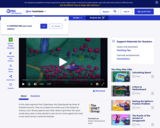Educational Use
Rating
0.0 stars

The CyberSquad tests which broom can travel the furthest in five seconds in this video from Cyberchase.

Subject:
Mathematics
Physical Science
Physics
Material Type:
Lecture
Provider:
PBS LearningMedia
Provider Set:
PBS Learning Media: Multimedia Resources for the Classroom and Professional Development
Author:
U.S. Department of Education
WNET
07/08/2008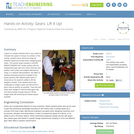Educational Use
Rating
0.0 stars

A gear is a simple machine that is very useful to increase the speed or torque of a wheel. In this activity, students learn about the trade-off between speed and torque when designing gear ratios. The activity setup includes a LEGO(TM) MINDSTORMS(TM) NXT pulley system with two independent gear sets and motors that spin two pulleys. Each pulley has weights attached by string. In a teacher demonstration, the effect of adding increasing amounts of weight to the pulley systems with different gear ratios is observed as the system's ability to lift the weights is tested. Then student teams are challenged to design a gear set that will lift a given load as quickly as possible. They test and refine their designs to find the ideal gear ratio, one that provides enough torque to lift the weight while still achieving the fastest speed possible.

Subject:
Applied Science
Engineering
Material Type:
Activity/Lab
Provider:
TeachEngineering
Provider Set:
TeachEngineering
Author:
Jeffrey Laut
Paul Phamduy
10/14/2015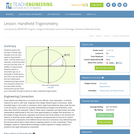Educational Use
Rating
0.0 stars

Students explore the concept of similar right triangles and how they apply to trigonometric ratios. Use this lesson as a refresher of what trig ratios are and how they work. In addition to trigonometry, students explore a clinometer app on an Android® or iOS® device and how it can be used to test the mathematics underpinning trigonometry. This prepares student for the associated activity, during which groups each put a clinometer through its paces to better understand trigonometry.

Subject:
Applied Science
Engineering
Mathematics
Trigonometry
Material Type:
Lesson Plan
Provider:
TeachEngineering
Provider Set:
TeachEngineering
Author:
Scott Burns
09/18/2014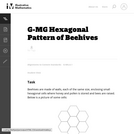Unrestricted Use
CC BY
Rating
0.0 stars

The goal of this task is to use geometry study the structure of beehives. Beehives have a tremendous simplicity as they are constructed entirely of small, equally sized walls. In order to as useful as possible for the hive, the goal should be to create the largest possible volume using the least amount of materials. In other words, the ratio of the volume of each cell to its surface area needs to be maximized. This then reduces to maximizing the ratio of the surface area of the cell shape to its perimeter.

Subject:
Geometry
Mathematics
Material Type:
Activity/Lab
Provider:
Illustrative Mathematics
Provider Set:
Illustrative Mathematics
Author:
Illustrative Mathematics
01/21/2013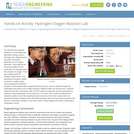Educational Use
Rating
0.0 stars

This lab exercise exposes students to a potentially new alternative energy source hydrogen gas. Student teams are given a hydrogen generator and an oxygen generator. They balance the chemical equation for the combustion of hydrogen gas in the presence of oxygen. Then they analyze what the equation really means. Two hypotheses are given, based on what one might predict upon analyzing the chemical equation. Once students have thought about the process, they are walked through the experiment and shown how to collect the gas in different ratios. By trial and error, students determine the ideal combustion ratio. For both volume of explosion and kick generated by explosion, they qualitatively record results on a 0-4 scale. Then, students evaluate their collected results to see if the hypotheses were correct and how their results match the theoretical equation. Students learn that while hydrogen will most commonly be used for fuel cells (no combustion situation), it has been used in rocket engines (for which a tremendous combustion occurs).

Subject:
Applied Science
Chemistry
Engineering
Physical Science
Material Type:
Activity/Lab
Provider:
TeachEngineering
Provider Set:
TeachEngineering
Author:
Courtney Herring
Stephen Dent
09/18/2014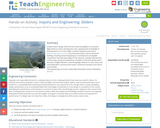Educational Use
Rating
0.0 stars

Student teams design, build and test small-sized gliders to maximize flight distance and an aerodynamic ratio, applying their knowledge of fluid dynamics to its role in flight. Students experience the entire engineering design process, from brainstorming to CAD (or by hand) drafting, including researching (physics of aerodynamics and glider components that take advantage of that science), creating materials lists, constructing, testing and evaluating—all within constraints (works with a launcher, budget limitation, maximizing flight distance to mass ratio), and concluding with a summary final report. Numerous handouts and rubrics are provided.

Subject:
Applied Science
Engineering
Mathematics
Measurement and Data
Physical Science
Physics
Material Type:
Activity/Lab
Provider:
TeachEngineering
Provider Set:
Activities
Author:
Melanie Finn-Scofield
01/01/2015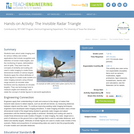Educational Use
Rating
0.0 stars

Students learn about radar imaging and its various military and civilian applications that include recognition and detection of human-made targets, and the monitoring of space, deforestation and oil spills. They learn how the concepts of similarity and scaling are used in radar imaging to create three-dimensional models of various targets. Students apply the critical attributes of similar figures to create scale models of a radar imaging scenario using infrared range sensors (to emulate radar functions) and toy airplanes (to emulate targets). They use technology tools to measure angles and distances, and relate the concept of similar figures to real-world applications.

Subject:
Education
Material Type:
Activity/Lab
Provider:
TeachEngineering
Provider Set:
TeachEngineering
Author:
Mounir Ben Ghalia
Rocio Denise Nava
10/14/2015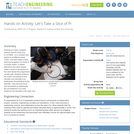Educational Use
Rating
0.0 stars

Working as a team, students discover that the value of pi (3.1415926...) is a constant and applies to all different sized circles. The team builds a basic robot and programs it to travel in a circular motion. A marker attached to the robot chassis draws a circle on the ground as the robot travels the programmed circular path. Students measure the circle's circumference and diameter and calculate pi by dividing the circumference by the diameter. They discover the pi and circumference relationship; the circumference of a circle divided by the diameter is the value of pi.

Subject:
Applied Science
Computing and Information
Engineering
Mathematics
Technology
Material Type:
Activity/Lab
Provider:
TeachEngineering
Provider Set:
TeachEngineering
Author:
Carole Chen
Michael Hernandez
09/18/2014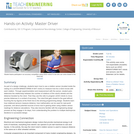Educational Use
Rating
0.0 stars

As part of a design challenge, students learn how to use a rotation sensor (located inside the casing of a LEGO® MINDSTORMS ® NXT motor) to measure how far a robot moves with each rotation. Through experimentation and measurement with the sensor, student pairs determine the relationship between the number of rotations of the robot's wheels and the distance traveled by the robot. Then they use this ratio to program LEGO robots to move precise distances in a contest of accuracy. The robot that gets closest to the goal without touching the toy figures at the finish line is the winning programming design. Students learn how rotational sensors measure distance, how mathematics can be used for real-world purposes, and about potential sources of error due to gearing when using rotation sensor readings for distance calculations. They also become familiar with the engineering design process as they engage in its steps, from understanding the problem to multiple test/improve iterations to successful design.

Subject:
Applied Science
Career and Technical Education
Electronic Technology
Engineering
Material Type:
Activity/Lab
Provider:
TeachEngineering
Provider Set:
TeachEngineering
Author:
Nishant Sinha
Pranit Samarth
Satish S. Nair
09/18/2014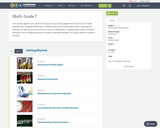Conditional Remix & Share Permitted
CC BY-NC
Rating
0.0 stars

Four full-year digital course, built from the ground up and fully-aligned to the Common Core State Standards, for 7th grade Mathematics. Created using research-based approaches to teaching and learning, the Open Access Common Core Course for Mathematics&nbsp;is designed with student-centered learning in mind, including activities for students to develop valuable 21st century skills and academic mindset.

Subject:
Mathematics
Material Type:
Full Course
Provider:
Pearson
10/06/2016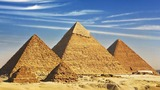Conditional Remix & Share Permitted
CC BY-NC
Rating
0.0 stars

Zooming In On Figures

Unit Overview

Type of Unit: Concept; Project

Length of Unit: 18 days and 5 days for project

Prior Knowledge

Students should be able to:

Find the area of triangles and special quadrilaterals.
Use nets composed of triangles and rectangles in order to find the surface area of solids.
Find the volume of right rectangular prisms.
Solve proportions.

Lesson Flow

After an initial exploratory lesson that gets students thinking in general about geometry and its application in real-world contexts, the unit is divided into two concept development sections: the first focuses on two-dimensional (2-D) figures and measures, and the second looks at three-dimensional (3-D) figures and measures.
The first set of conceptual lessons looks at 2-D figures and area and length calculations. Students explore finding the area of polygons by deconstructing them into known figures. This exploration will lead to looking at regular polygons and deriving a general formula. The general formula for polygons leads to the formula for the area of a circle. Students will also investigate the ratio of circumference to diameter ( pi ). All of this will be applied toward looking at scale and the way that length and area are affected. All the lessons noted above will feature examples of real-world contexts.
The second set of conceptual development lessons focuses on 3-D figures and surface area and volume calculations. Students will revisit nets to arrive at a general formula for finding the surface area of any right prism. Students will extend their knowledge of area of polygons to surface area calculations as well as a general formula for the volume of any right prism. Students will explore the 3-D surface that results from a plane slicing through a rectangular prism or pyramid. Students will also explore 3-D figures composed of cubes, finding the surface area and volume by looking at 3-D views.
The unit ends with a unit examination and project presentations.

Subject:
Geometry
Mathematics
Material Type:
Unit of Study
Provider:
Pearson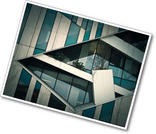Conditional Remix & Share Permitted
CC BY-NC
Rating
0.0 stars

Gallery OverviewAllow students who have a clear understanding of the content thus far in the unit to work on Gallery problems of their choosing. You can then use this time to provide additional help to students who need review of the unit&rsquo;s concepts or to assist students who may have fallen behind on work.Problem DescriptionsSprinklersExplore different sprinkler layouts, looking at circular areas (and partial circles) to decide which will be best to water a lawn.Leaning TowerChoose a scale and use a ruler and protractor to make a simple scale drawing.Pizza DoublerIf you could choose between doubling the fraction of the pizza that a slice is, or doubling the radius, which option would give you more pizza? In this problem you will investigate which choice gives a bigger slice.Area and ScaleWhen a figure is redrawn at a larger scale the side lengths increase by the factor of the scale (if the scale doubles the size, the side lengths double also). But, does the area increase the same way? Explore a dynamic sketch and see how area changes when the scale changes.Tree House 1Given plans for a tree house, redraw the plans at a different scale.

Subject:
Geometry
Material Type:
Lesson Plan
09/21/2015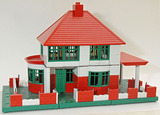Conditional Remix & Share Permitted
CC BY-NC
Rating
0.0 stars

Students will explore scale and use it to find measurements in scale drawings.Key ConceptsScale drawings are drawn proportionally so that there is a ratio between a given length on the drawing and the actual length. This ratio is used to set up a proportion to find other measurements.GoalsUnderstand that scale drawings are proportional.Use scale to find actual measurements.ELL: Define these terms in the context of the discussion:scalescale drawingscaled to fitproportionalAllow ELLs to use the dictionary if they wish.

Subject:
Geometry
Ratios and Proportions
Material Type:
Lesson Plan